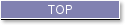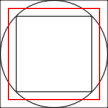## LIBER CHAOS Bl. Raymond Lull Doctor Illuminatus

###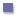Part I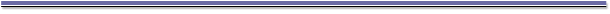###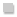The Four Degrees of the Elements in Chaos

1. In the first degree of Chaos, God established in potentiality the degrees of animal, vegetal and mineral species, in the second He brought their degrees into act by creating them, so that from the second to the third there are four degrees of elements proceeding from the generating agent.

2. As there are four elements, God established four degrees for the quality of each, as for instance in the heat of fire: now some plants are hot in the fourth degree, some in the third, some in the second and some in the first. A herb or plant which is hot in the fourth degree, is dry in the third degree, because fire is hot per se and dry by accident; and this plant is moist in the second degree since fire is in concordance with air as it gives it heat. But because fire is opposed to water, a herb which is hot in the fourth degree must be cold in the first, and thus the said herb has four degrees: at the head is fire in the fourth degree of heat, followed by earth in the third degree of dryness, then air in the second degree of moisture and finally water in the first degree of cold.

3. In the said herb, fire divides itself into six points as follows: fire gives three points of heat to the three points of earth's dryness, and it gives two points of heat to the two points of moisture, and one point of heat to the point of cold; and this is how the degrees are proportioned and quantified in this supposite or herb which contains twelve points in itself, as we said.

4. This kind of supposite, which is hot in the fourth degree, is composed, as described, of twelve points, six of which belong to fire and the other six to the other elements, these are divided into three points of earth, two of air and one of water. The supposite moreover retains for itself a seventh point of heat which is simple and rules the six points of heat present in the supposite with the other elements' points, and it also retains a fourth point of dryness which rules the three points of dryness, a third point of moisture which rules the two points of moisture, and likewise a second point of cold which rules the single point of cold. This shows how the elements in the said supposite which is in the fourth degree of heat are both simple and compound: now the seventh point of heat, the fourth of dryness, the third of moisture and the second of cold are simple points which exist not only virtually, but even essentially within and outside the said supposite and which, as ordered in the second degree of Chaos, instill from the first degree of Chaos into the third, the degrees in the said supposite, namely the fourth, third, second and first as they exist in composition, mixture and digestion in the supposite, and their subject is the essence of the same supposite existing in the third degree of Chaos.

5. When the said supposite decays and its numerical identity is annihilated by corruption, then its fourth degree of heat which previously existed in the third degree of Chaos, reverts to the first degree of Chaos by means of this seventh point, and the third degree of dryness does the same through the fourth point, and the other elements do likewise, whereupon the subject of the four degrees reverts from the third degree of Chaos to the first, and this is enough about all species hot in the fourth degree.

6. Now let us look at a herb of the species which is hot in the third degree. Every elemented species which is hot in the third degree is dry in the second, moist in the first, and cold is downgraded in this species because it cannot have a whole degree of cold for itself. And likewise, as described above, in this species which is hot in the third degree, fire gives two points of heat to two points of dryness, one point of heat to one point of moisture and three quarters of one point of heat to the three quarters of a point of cold present in this species. Thus, the supposite contains seven and a half points: of these, three and three quarters belong to fire and the remainder to the other elements, and the supposite retains for itself a fourth point of heat which is simple and rules these three points and the other points of heat which are in composition; it also retains a third point of dryness which is simple and rules the two points of compound dryness, and likewise, it retains a second simple point of moisture which rules one point of compound moisture, and it also retains in this way a first point of cold which is simple and rules three quarters of one compound point. This can be likewise understood in the same order with regard to species in the second and first degrees of heat, so that we need not dwell any longer on this here, because what was said also applies to the fourth, third, second and first degrees of the other complexions.